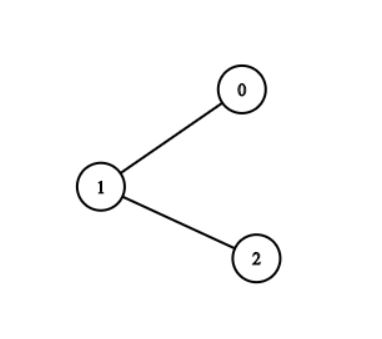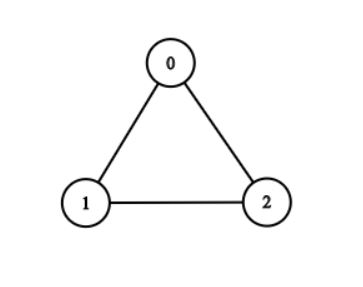New update is available. Click here to update.

# Is It A Tree?

Contributed by
Shivam Kumar Singh
Last Updated: 23 Feb, 2023
Medium0/80
Avg time to solve 20 mins
Success Rate 75 %Share21 upvotes

## Problem Statement

Suggest Edit

#### Given a graph with 'V' vertices numbered from 0 to 'V' - 1 and 'E' edges. Determine if it is a tree or not?

Detailed explanation ( Input/output format, Notes, Images )##### Constraints :
``````1 < 'V' <= 10^5
0 <= 'E' <= min(10^5, V*(V-1)/2)
0 <= u, v <= V-1

Time Limit: 1 sec
``````
##### Sample Input 1:
``````3 2
0 1
1 2
``````
##### Sample Output 1:
``````True
``````
##### Explanation of Sample Input 1:
``````We clearly can see that it is a tree since it satisfies the property of a tree.
``````##### Sample Input 2:
``````3 3
0 1
1 2
0 2
``````
##### Sample Output 2:
``````False
``````
##### Explanation of Sample Input 2:
``````As we can see that it is not a tree since it doesn't satisfy the property of a tree.
``````AutoConsole